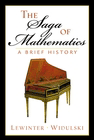#### The Saga of Mathematics: A Brief History

Martin Lewinter
William Widulski

Prentice Hall
ISBN: 0-13-034079-0### Errata

1. On page 141, the word "predicable" should be spelled "predictable" in the paragraph that starts "Aha!"
2. On page 191, Figure 10-1 is missing a bridge connecting the islands "B" and "D".
3. On page 228, Exercise 1 should read "Let u = [2, −2, 2], v = [1, 2, 0] and w = [−3, 2, −2]. Find ..."
4. On page 250, line 2, should read "In the equation 2x2 + 3x − 5 = 0, ..."
5. On page 259, The first paragrph of Example 13-5 should read "The LCD of the fractions is 2x, so we multiply both sides of the equation by 2x, remembering to multiply the 2x to both fractions using the distributive law, yielding ..."
6. On page 261, Exercise 3 should read "Set each of the quadratic expressions in Exercise 2 equal to y and graph the resulting equation. Be sure ..."
7. On page 272, 10 (b) should be 9/46 = 1/6 + 1/46 + 1/138
8. On page 272, 11 (d) should be 7/50 = 1/10 + 1/25